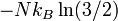# Entropy of ice phases

The ice rules, also known as the Bernal-Fowler rules , describe how the hydrogen atoms are distributed in the ices. Each oxygen atom has two hydrogen atoms attached to it, at a distance of approximately 1 ångström, one hydrogen atom resides on each O-O bond. There are many ways to distribute the protons such that these rules are satisfied, and all are equally probable. For this reason, the residual entropy of ice is correctly predicted. The observed residual entropy was described for the first time using the statistical model for ice Ih introduced by Linus Pauling . Pauling suggested a random arrangement of protons. By means of a simple calculation he showed that the resulting disordered phase requires the addition of a combinatorial entropy of$-Nk_B \ln (3/2)$ to the theoretical estimate. This finding demonstrated that a crystal phase such as ice Ih could show full disorder at 0K, which is in contrast to the prediction from the third principle of thermodynamics.